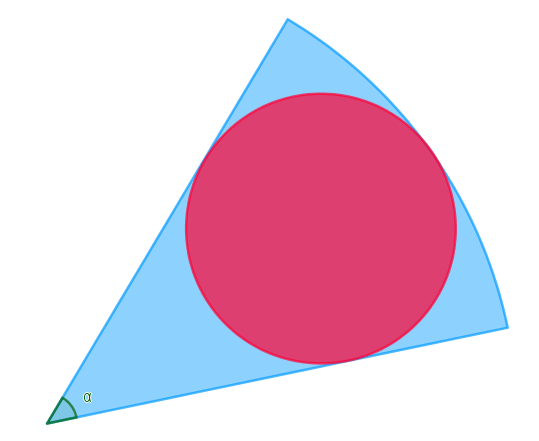# Piece of cake ?

Calculus Level 5

The red circle is the biggest circle inscribed in the circular sector of angle $\alpha$ and radius $100$.If $\alpha$ is chosen uniformly at random between $0^\circ$ and $180^\circ$, what is the expected value of the radius of the red circle? (Give your result to 3 decimals.)

×

Problem Loading...

Note Loading...

Set Loading...